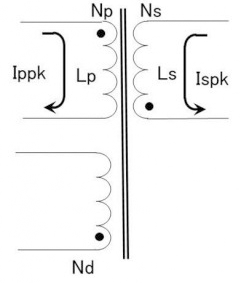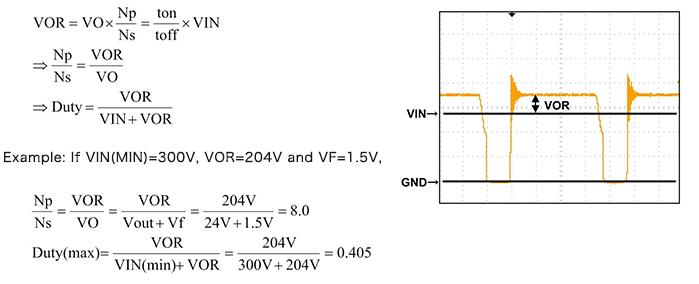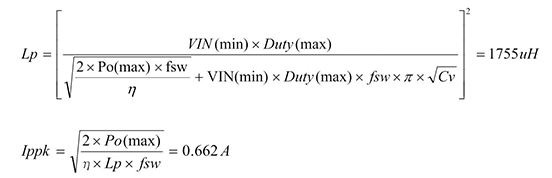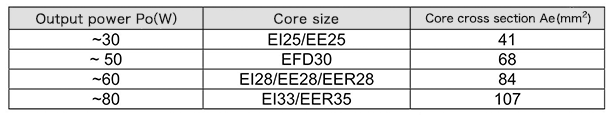Technical Information Site of Power Supply Design

• Basic Knowledge
• AC/DC
• Design Example of Isolated Quasi-Resonant Converters Using SiC MOSFET
• Transformer T1 Design - 1

2018.08.09 AC/DC

# Transformer T1 Design - 1

Design Example of Isolated Quasi-Resonant Converters Using SiC MOSFET

From this session, we begin calculations of component values and other factors and detailed circuit design, beginning with the design of a transformer T1. Calculation procedures are described below. This is substantially the same approach as ”Designing Isolated Flyback Converter Circuits: Transformer Design (Calculating Numerical Values) “, and therefore the latter should be reviewed as well.

• ①Setting a flyback voltage VOR
• ②Calculating the primary winding inductance Lp and primary-side peak current Ippk
• ③Determining the transformer size
• ④Calculating the primary winding turns Np
• ⑤Calculating the secondary winding turns Ns
• ⑥Calculating the VCC winding turns NdParameters that must be derived for the transformer design are the core size, the inductance Lp, and the winding turns Np, Ns, Nd.

In addition, conditions imposed on the transformer T1 are an output of 24 V at 1 A, and VIN(DC) of from 300 V to 900 V.

The previously presented circuit diagram should be consulted as necessary.

①Setting a flyback voltage VOR

The flyback voltage VOR is equal to VO (the secondary Vout plus the VF for the secondary diode DN1) multiplied by the transformer winding ratio Np:Ns. Setting the flyback voltage VOR determines the winding ratio Np：Ns and the duty ratio. The basic equation and an example are given below. (DN1 does not appear in the circuit diagram, but the two diodes connected on the secondary side of T1 are DN1.)VOR should be set so that the duty is 0.5 or lower, in consideration of MOSFET losses and the like. The graph shows the MOSFET Vds waveform.

②Calculating the primary winding inductance Lp and primary-side peak current Ippk

The minimum oscillation frequency fsw at minimum input voltage (VIN=300 V) and maximum load is determined, and the primary winding inductance Lp and primary-side peak current Ippk are calculated.

We set the minimum oscillation frequency at minimum input voltage (VIN=300 V) to fsw=92 kHz. The other parameters are determined as follows.

• ・From Po=24V X 1A=24W, and considering overload protection and the like, Po(max)=30 W（derating: 0.8）
• ・Transformer efficiency η=85%
• ・Resonant capacitance Cv=100pF③Determining the transformer core size

Given Po(max)=30 W, a moderate margin is included to select the EFD30 core size. The following table lists core sizes that are regarded as suitable for a given output power Po. The values in the table below only represent rough approximations for output power Po. For details, transformer manufacturers should be consulted.Thus the required core size and inductance Lp have been determined. The winding turns Np, Ns, Nd will be calculated in the next section.

Parameters Necessary for Transformer Design

Transformer core size EFD30 or compatible 1750µH (to be calculated in the next section) (to be calculated in the next section) (to be calculated in the next section)

#### Key Points:

・The core size, primary inductance and number of turns for a transformer T1 are calculated according to the procedure described.

・Calculations can be performed according to more or less the same approach as in “Designing Isolated Flyback Converter Circuits: Transformer Design (Calculating Numerical Values)”.